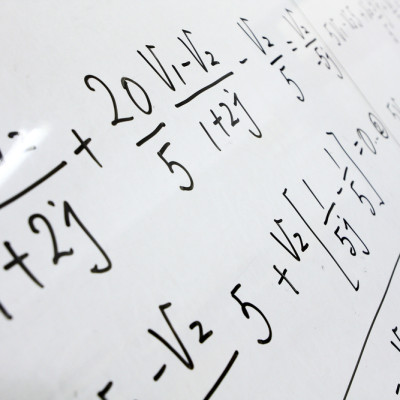# Day 6: A Fun Game

Probability Level 5A mathematician writes the integers $1,2,3,4,\ldots,2015$ on a whiteboard, with which he plays a game.

Every turn he selects two numbers $a$ and $b$ that are on the whiteboard, subject to two conditions:

• If one of $a$ and $b$ are prime, he will always let $a$ be prime; so if he picks 5 and 8, then he will let $a = 5, b= 8$.
• But if both are prime, or both are not prime, then $a \leq b$

Then he rubs off $a$ and $b$ and replaces them with $a^2b+2a-4b-2$.

He continues this process until one number is left on the whiteboard.

What number is this?

Note: By primes I do only mean positive primes only.

×